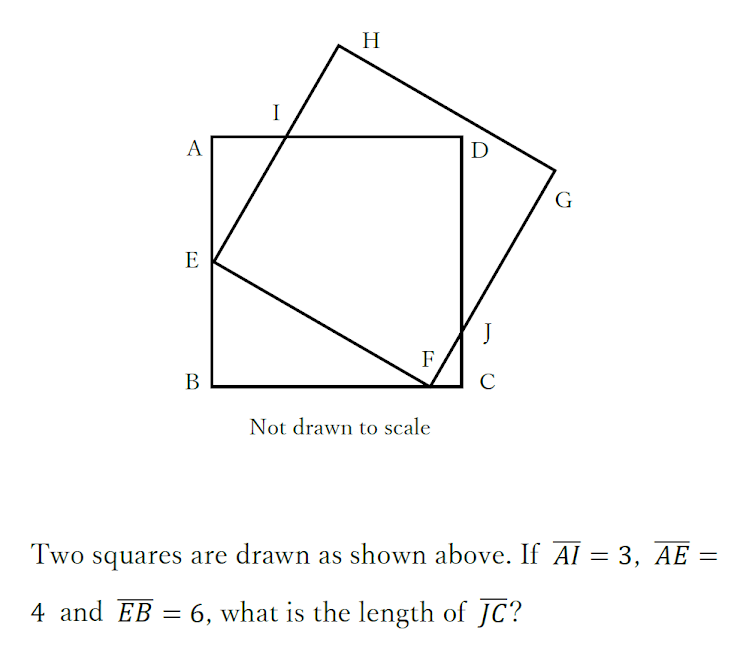SAT攻略講座 第2回 Section4 Calculator
Calculator 15 min

Q1
1 point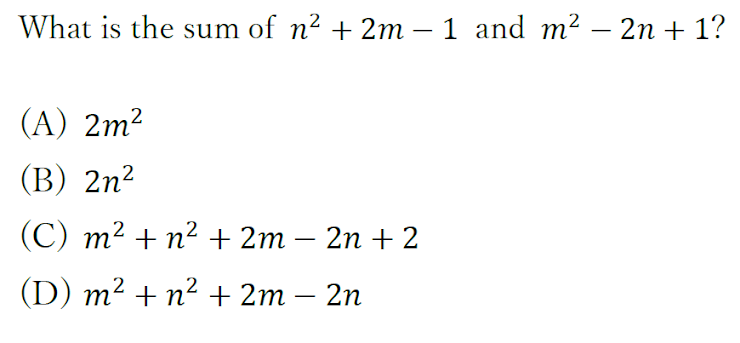Q2
1 point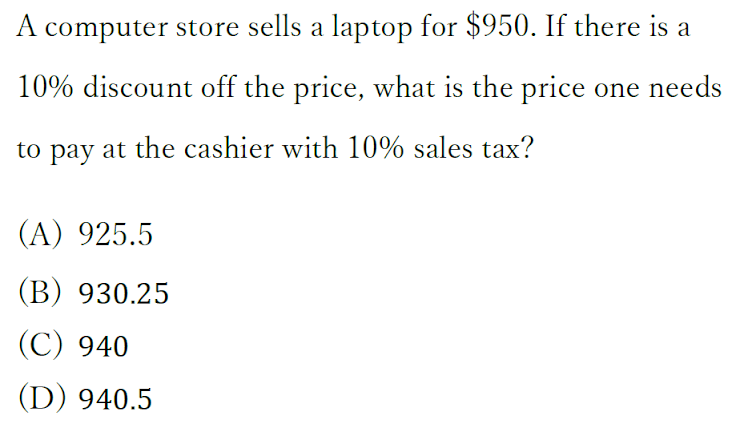Q3
1 point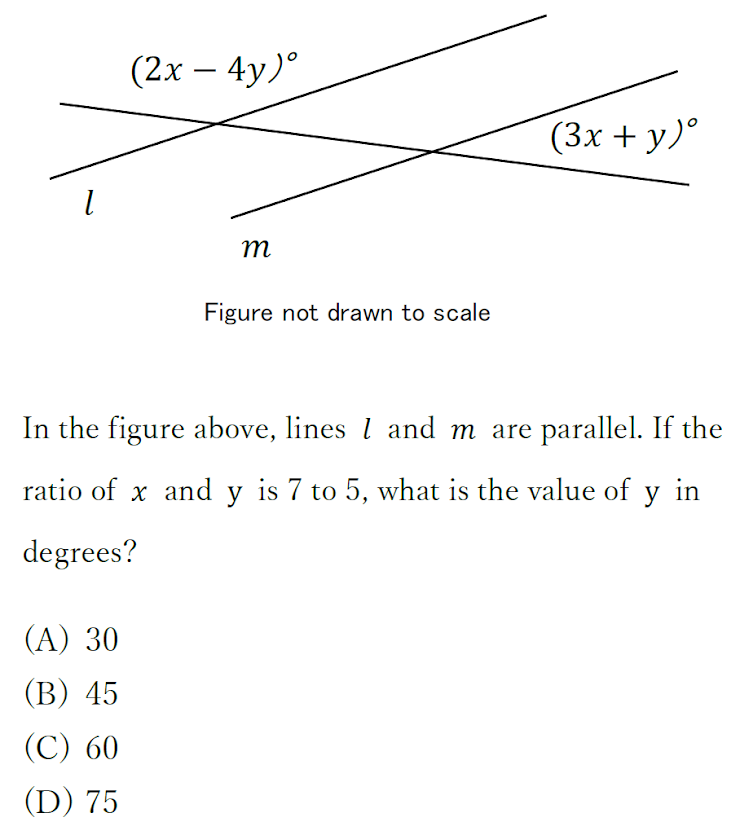Q4
1 point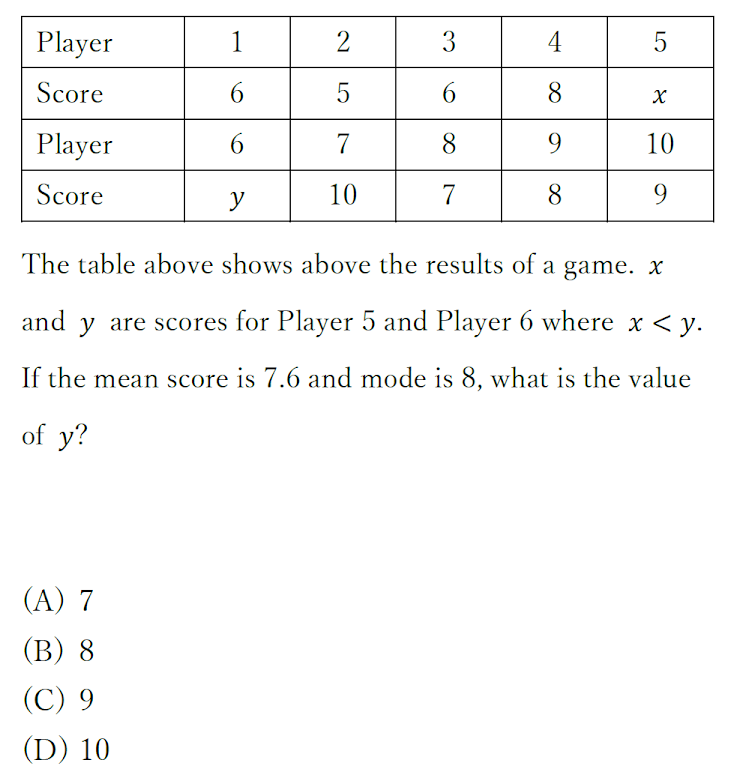Q5
1 point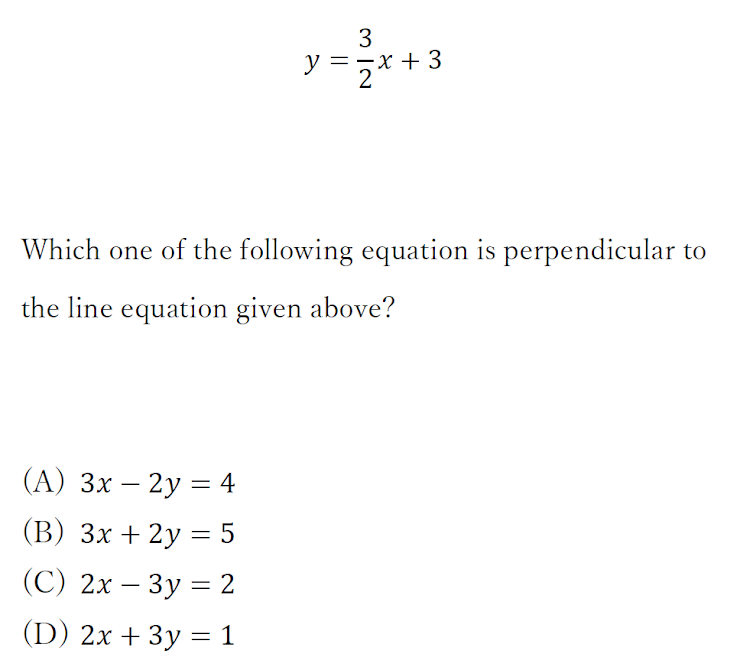Q6 and Q7 refere to the following information.
1 point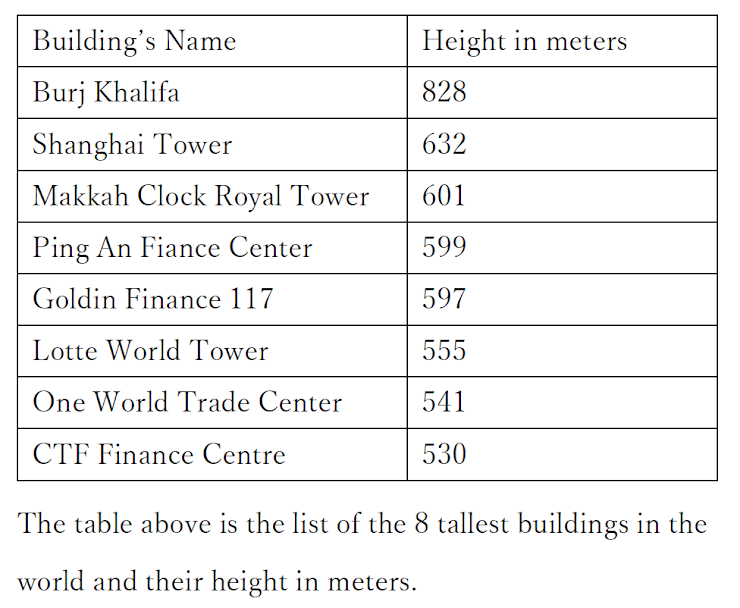What is the arithmetic mean of the height in meters of the eight buildings shown above?
Q7
1 point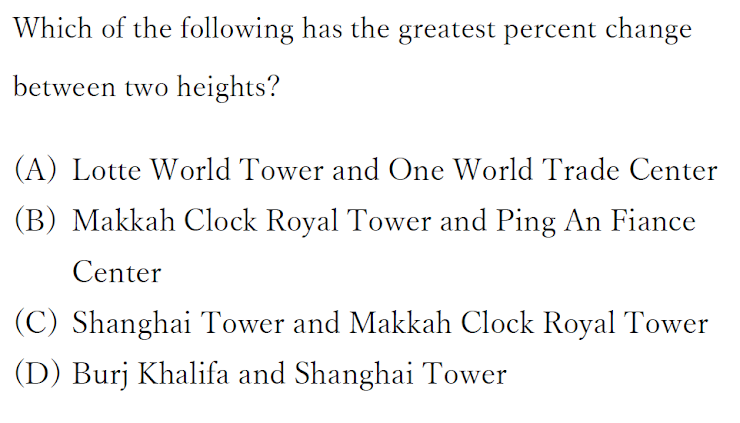Q8
1 point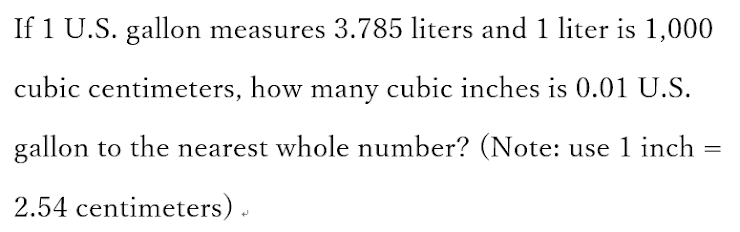Q9
1 pointQ10
1 point